# Atomic structure

1 de Jul de 2013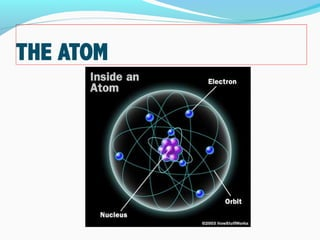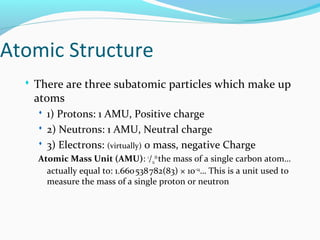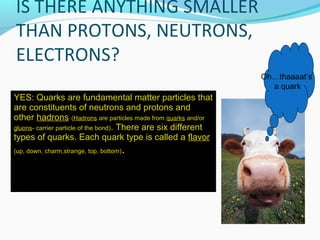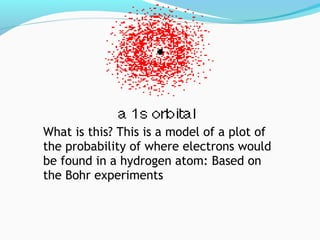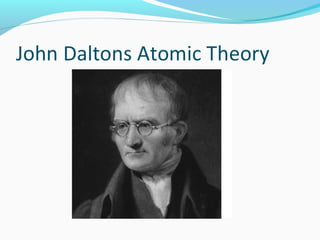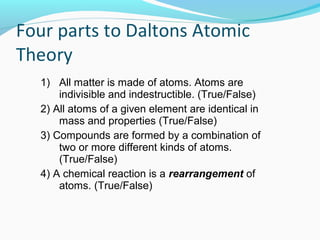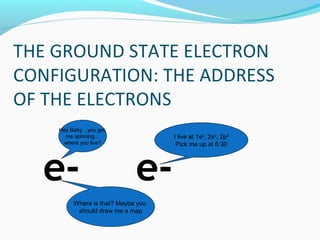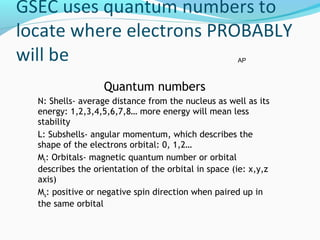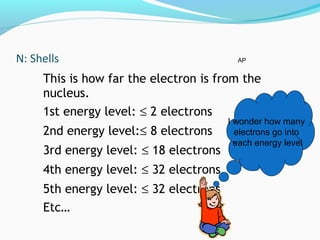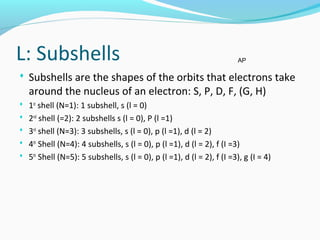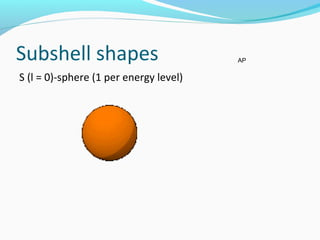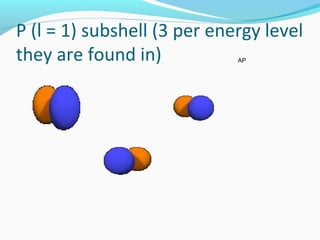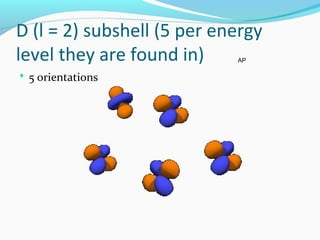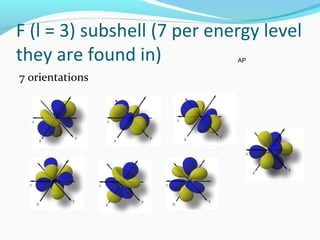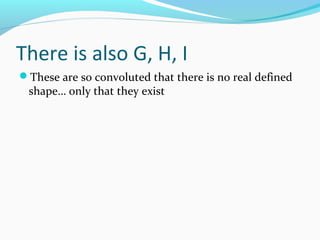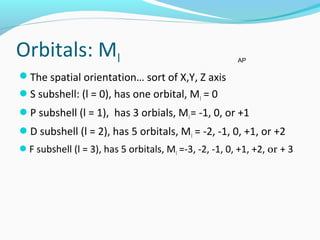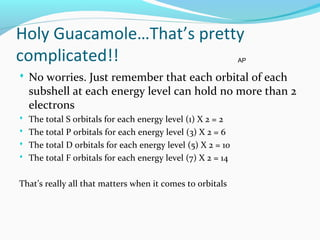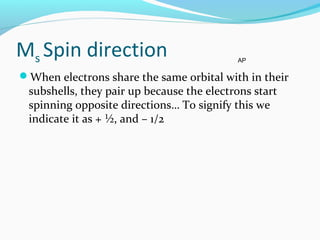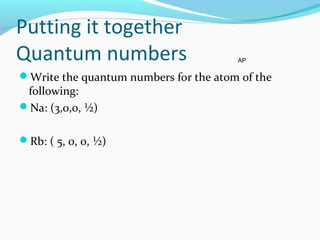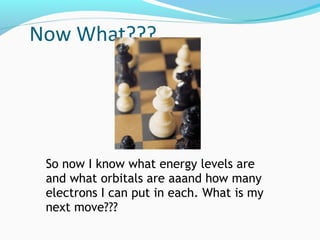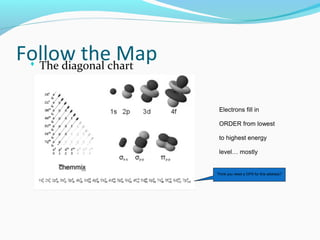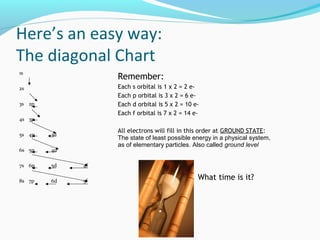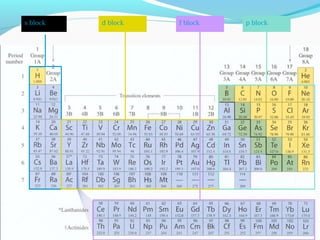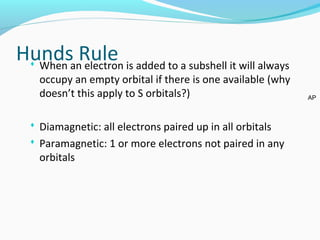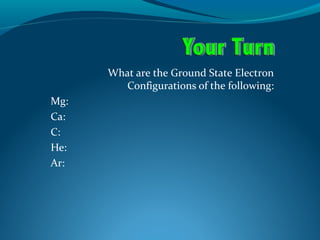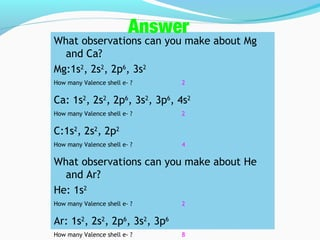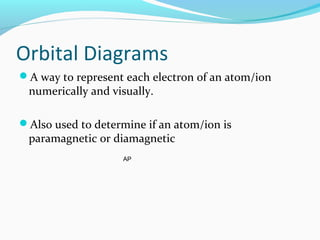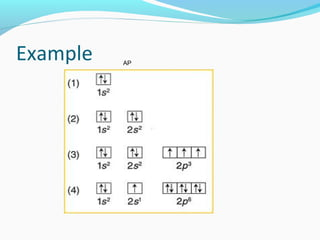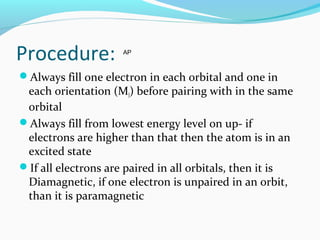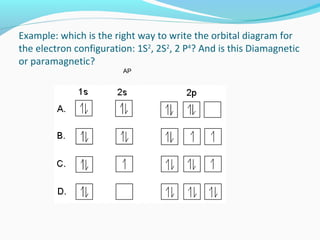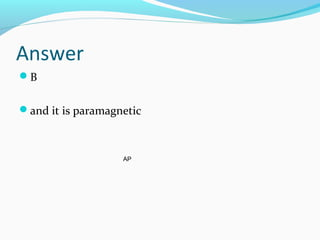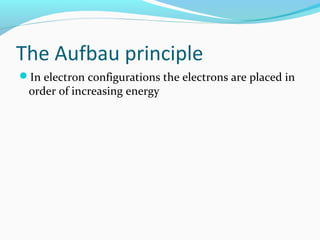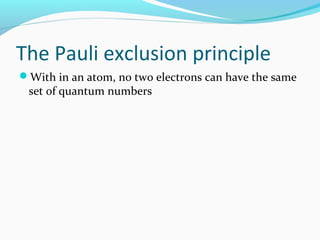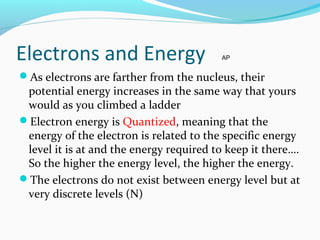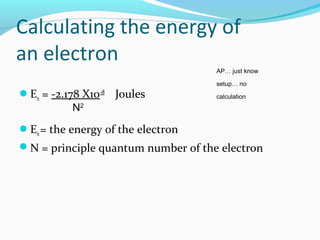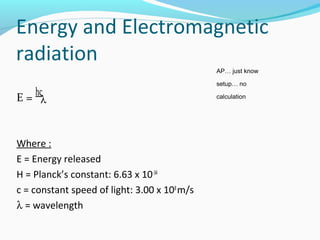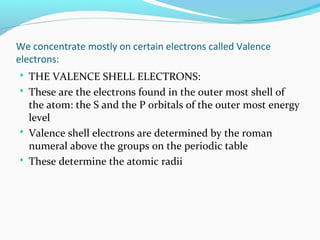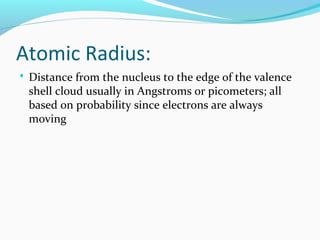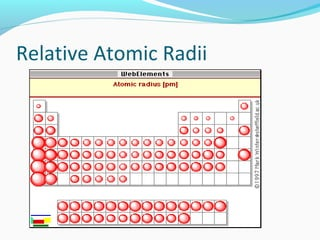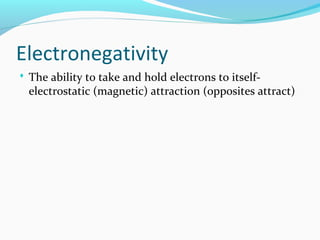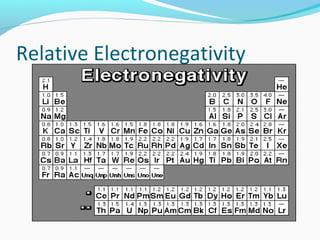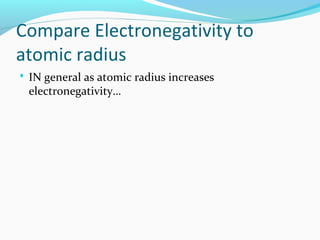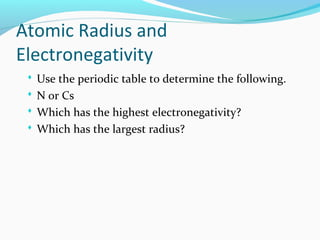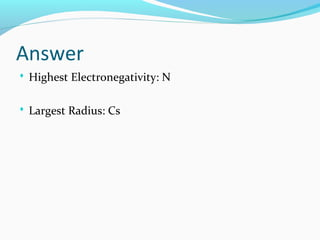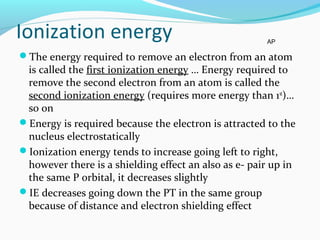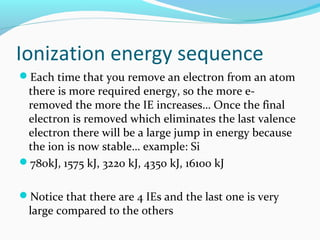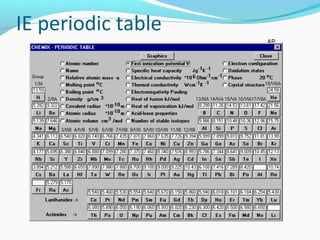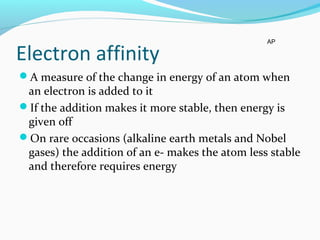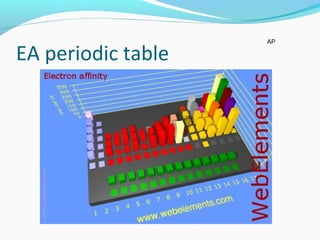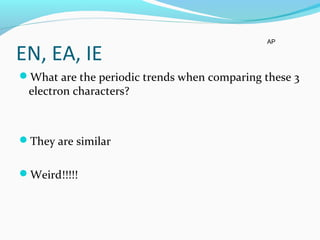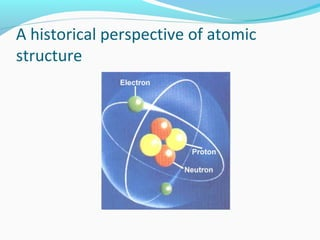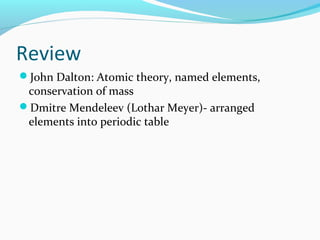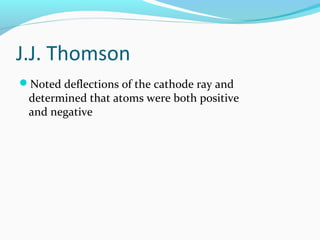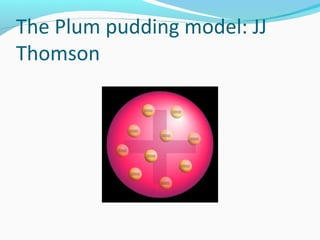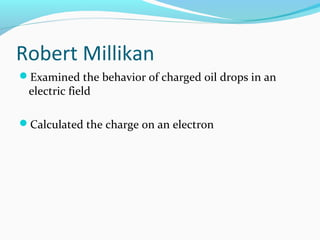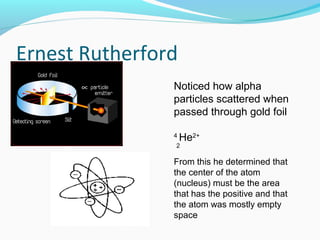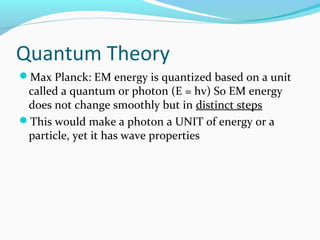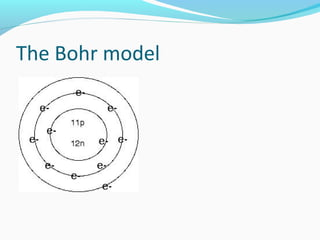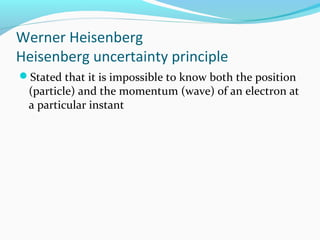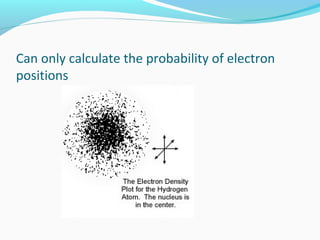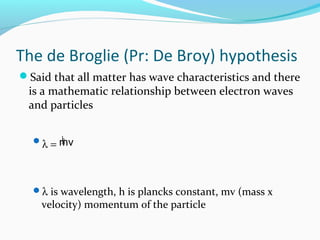1 de 66

### Atomic structure

• 2. Atomic Structure  There are three subatomic particles which make up atoms  1) Protons: 1 AMU, Positive charge  2) Neutrons: 1 AMU, Neutral charge  3) Electrons: (virtually) 0 mass, negative Charge Atomic Mass Unit (AMU): 1 /12 th the mass of a single carbon atom… actually equal to: 1.660538782(83) × 10    −24 … This is a unit used to measure the mass of a single proton or neutron
• 3. YES: Quarks are fundamental matter particles that are constituents of neutrons and protons and other hadrons (Hadrons are particles made from quarks and/or gluons- carrier particle of the bond). There are six different types of quarks. Each quark type is called a flavor (up, down, charm,strange, top, bottom). Oh…thaaaat’s a quark
• 4. What is this? This is a model of a plot of the probability of where electrons would be found in a hydrogen atom: Based on the Bohr experiments
• 5. QuickTime™ and a TIFF (Uncompressed) decompressor are needed to see this picture.
• 6. 1) All matter is made of atoms. Atoms are indivisible and indestructible. (True/False) 2) All atoms of a given element are identical in mass and properties (True/False) 3) Compounds are formed by a combination of two or more different kinds of atoms. (True/False) 4) A chemical reaction is a rearrangement of atoms. (True/False)
• 7. e- e- Hey Baby…you get me spinning… where you live? I live at 1s2 , 2s2 , 2p5 Pick me up at 6:30 Where is that? Maybe you should draw me a map
• 8. Quantum numbers N: Shells- average distance from the nucleus as well as its energy: 1,2,3,4,5,6,7,8… more energy will mean less stability L: Subshells- angular momentum, which describes the shape of the electrons orbital: 0, 1,2… Ml: Orbitals- magnetic quantum number or orbital describes the orientation of the orbital in space (ie: x,y,z axis) Ms: positive or negative spin direction when paired up in the same orbital AP
• 9. This is how far the electron is from the nucleus. 1st energy level: ≤ 2 electrons 2nd energy level:≤ 8 electrons 3rd energy level: ≤ 18 electrons 4th energy level: ≤ 32 electrons 5th energy level: ≤ 32 electrons Etc… I wonder how many electrons go into each energy level AP
• 10. L: Subshells  Subshells are the shapes of the orbits that electrons take around the nucleus of an electron: S, P, D, F, (G, H)  1st shell (N=1): 1 subshell, s (l = 0)  2nd shell (=2): 2 subshells s (l = 0), P (l =1)  3rd shell (N=3): 3 subshells, s (l = 0), p (l =1), d (l = 2)  4th Shell (N=4): 4 subshells, s (l = 0), p (l =1), d (l = 2), f (I =3)  5th Shell (N=5): 5 subshells, s (l = 0), p (l =1), d (l = 2), f (I =3), g (I = 4) AP
• 11. Orbitals
• 12. Subshell shapes S (l = 0)-sphere (1 per energy level) AP
• 13. P (l = 1) subshell (3 per energy level they are found in) AP
• 14. D (l = 2) subshell (5 per energy level they are found in)  5 orientations AP
• 15. F (l = 3) subshell (7 per energy level they are found in) 7 orientations AP
• 16. There is also G, H, I These are so convoluted that there is no real defined shape… only that they exist
• 17. How does the l relate to Ml? It is all about orientation
• 18. Orbitals: Ml The spatial orientation… sort of X,Y, Z axis S subshell: (l = 0), has one orbital, Ml = 0 P subshell (l = 1), has 3 orbials, Ml= -1, 0, or +1 D subshell (l = 2), has 5 orbitals, Ml = -2, -1, 0, +1, or +2 F subshell (l = 3), has 5 orbitals, Ml =-3, -2, -1, 0, +1, +2, or + 3 AP
• 19. Holy Guacamole…That’s pretty complicated!!  No worries. Just remember that each orbital of each subshell at each energy level can hold no more than 2 electrons  The total S orbitals for each energy level (1) X 2 = 2  The total P orbitals for each energy level (3) X 2 = 6  The total D orbitals for each energy level (5) X 2 = 10  The total F orbitals for each energy level (7) X 2 = 14 That’s really all that matters when it comes to orbitals AP
• 20. Ms Spin direction When electrons share the same orbital with in their subshells, they pair up because the electrons start spinning opposite directions… To signify this we indicate it as + ½, and – 1/2 AP
• 21. Putting it together Quantum numbers Write the quantum numbers for the atom of the following: Na: (3,0,0, ½) Rb: ( 5, 0, 0, ½) AP
• 22. Now What??? So now I know what energy levels are and what orbitals are aaand how many electrons I can put in each. What is my next move???
• 23. Follow the Map The diagonal chart Electrons fill in ORDER from lowest to highest energy level… mostly Think you need a GPS for this address?
• 24. Here’s an easy way: The diagonal Chart 1s 2s 3s 2p 4s 3p 5s 4p 3d 6s 5p 4d 7s 6p 5d 4f 8s 7p 6d 5f Remember: Each s orbital is 1 x 2 = 2 e- Each p orbital is 3 x 2 = 6 e- Each d orbital is 5 x 2 = 10 e- Each f orbital is 7 x 2 = 14 e- All electrons will fill in this order at GROUND STATE: The state of least possible energy in a physical system, as of elementary particles. Also called ground level What time is it?
• 25. Time to Hammer it home  H: 1s1  N: 1s2 ,2s2 , 2p4  Ne: 1s2 ,2s2 , 2p6
• 26. s block d block p blockf block
• 27. Hunds Rule When an electron is added to a subshell it will always occupy an empty orbital if there is one available (why doesn’t this apply to S orbitals?)  Diamagnetic: all electrons paired up in all orbitals  Paramagnetic: 1 or more electrons not paired in any orbitals AP
• 28. What are the Ground State Electron Configurations of the following: Mg: Ca: C: He: Ar:
• 29. What observations can you make about Mg and Ca? Mg:1s2 , 2s2 , 2p6 , 3s2 How many Valence shell e- ? 2 Ca: 1s2 , 2s2 , 2p6 , 3s2 , 3p6 , 4s2 How many Valence shell e- ? 2 C:1s2 , 2s2 , 2p2 How many Valence shell e- ? 4 What observations can you make about He and Ar? He: 1s2 How many Valence shell e- ? 2 Ar: 1s2 , 2s2 , 2p6 , 3s2 , 3p6 How many Valence shell e- ? 8 What observations can you make about Mg and Ca? Mg:1s2 , 2s2 , 2p6 , 3s2 How many Valence shell e- ? 2 Ca: 1s2 , 2s2 , 2p6 , 3s2 , 3p6 , 4s2 How many Valence shell e- ? 2 C:1s2 , 2s2 , 2p2 How many Valence shell e- ? 4 What observations can you make about He and Ar? He: 1s2 How many Valence shell e- ? 2 Ar: 1s2 , 2s2 , 2p6 , 3s2 , 3p6 How many Valence shell e- ? 8 Answer
• 30. Orbital Diagrams A way to represent each electron of an atom/ion numerically and visually. Also used to determine if an atom/ion is paramagnetic or diamagnetic AP
• 31. Example AP
• 32. Procedure: Always fill one electron in each orbital and one in each orientation (Ml) before pairing with in the same orbital Always fill from lowest energy level on up- if electrons are higher than that then the atom is in an excited state If all electrons are paired in all orbitals, then it is Diamagnetic, if one electron is unpaired in an orbit, than it is paramagnetic AP
• 33. Example: which is the right way to write the orbital diagram for the electron configuration: 1S2 , 2S2 , 2 P4 ? And is this Diamagnetic or paramagnetic? AP
• 34. Answer B and it is paramagnetic AP
• 35. The Aufbau principle In electron configurations the electrons are placed in order of increasing energy
• 36. The Pauli exclusion principle With in an atom, no two electrons can have the same set of quantum numbers
• 37. Electrons and Energy As electrons are farther from the nucleus, their potential energy increases in the same way that yours would as you climbed a ladder Electron energy is Quantized, meaning that the energy of the electron is related to the specific energy level it is at and the energy required to keep it there…. So the higher the energy level, the higher the energy. The electrons do not exist between energy level but at very discrete levels (N) AP
• 38. Calculating the energy of an electron En = -2.178 X10-18 Joules En= the energy of the electron N = principle quantum number of the electron N2 AP… just know setup… no calculation
• 39. Energy and Electromagnetic radiation E = hc Where : E = Energy released H = Planck’s constant: 6.63 x 10-34 c = constant speed of light: 3.00 x 108 m/s λ = wavelength λ AP… just know setup… no calculation
• 40. We concentrate mostly on certain electrons called Valence electrons:  THE VALENCE SHELL ELECTRONS:  These are the electrons found in the outer most shell of the atom: the S and the P orbitals of the outer most energy level  Valence shell electrons are determined by the roman numeral above the groups on the periodic table  These determine the atomic radii
• 41. Atomic Radius:  Distance from the nucleus to the edge of the valence shell cloud usually in Angstroms or picometers; all based on probability since electrons are always moving
• 42. Relative Atomic Radii QuickTime™ and a TIFF (Uncompressed) decompressor are needed to see this picture.
• 43. Electronegativity  The ability to take and hold electrons to itself- electrostatic (magnetic) attraction (opposites attract)
• 44. Relative Electronegativity QuickTime™ and a TIFF (Uncompressed) decompressor are needed to see this picture.
• 45. Compare Electronegativity to atomic radius  IN general as atomic radius increases electronegativity…
• 47. Atomic Radius and Electronegativity  Use the periodic table to determine the following.  N or Cs  Which has the highest electronegativity?  Which has the largest radius?
• 49. Ionization energy The energy required to remove an electron from an atom is called the first ionization energy … Energy required to remove the second electron from an atom is called the second ionization energy (requires more energy than 1st )… so on Energy is required because the electron is attracted to the nucleus electrostatically Ionization energy tends to increase going left to right, however there is a shielding effect an also as e- pair up in the same P orbital, it decreases slightly IE decreases going down the PT in the same group because of distance and electron shielding effect AP
• 50. Ionization energy sequence Each time that you remove an electron from an atom there is more required energy, so the more e- removed the more the IE increases… Once the final electron is removed which eliminates the last valence electron there will be a large jump in energy because the ion is now stable… example: Si 780kJ, 1575 kJ, 3220 kJ, 4350 kJ, 16100 kJ Notice that there are 4 IEs and the last one is very large compared to the others
• 51. IE periodic table AP
• 52. Electron affinity A measure of the change in energy of an atom when an electron is added to it If the addition makes it more stable, then energy is given off On rare occasions (alkaline earth metals and Nobel gases) the addition of an e- makes the atom less stable and therefore requires energy AP
• 53. EA periodic table AP
• 54. EN, EA, IE What are the periodic trends when comparing these 3 electron characters? They are similar Weird!!!!! AP
• 55. A historical perspective of atomic structure
• 56. Review John Dalton: Atomic theory, named elements, conservation of mass Dmitre Mendeleev (Lothar Meyer)- arranged elements into periodic table
• 57. J.J. Thomson Noted deflections of the cathode ray and determined that atoms were both positive and negative
• 58. The Plum pudding model: JJ Thomson
• 59. Robert Millikan Examined the behavior of charged oil drops in an electric field Calculated the charge on an electron
• 60. Ernest Rutherford Noticed how alpha particles scattered when passed through gold foil 4 He2+ From this he determined that the center of the atom (nucleus) must be the area that has the positive and that the atom was mostly empty space 2
• 61. Quantum Theory Max Planck: EM energy is quantized based on a unit called a quantum or photon (E = hv) So EM energy does not change smoothly but in distinct steps This would make a photon a UNIT of energy or a particle, yet it has wave properties
• 62. The Bohr model
• 63. Werner Heisenberg Heisenberg uncertainty principle Stated that it is impossible to know both the position (particle) and the momentum (wave) of an electron at a particular instant
• 64. Can only calculate the probability of electron positions
• 65. The de Broglie (Pr: De Broy) hypothesis Said that all matter has wave characteristics and there is a mathematic relationship between electron waves and particles λ = h λ is wavelength, h is plancks constant, mv (mass x velocity) momentum of the particle mv